# RD Sharma Solutions Class 9 Chapter 14 Quadrilaterals

Read RD Sharma Solutions Class 9 Chapter 14 Quadrilaterals below, students should study RD Sharma class 9 Mathematics available on Studiestoday.com with solved questions and answers. These chapter wise answers for class 9 Mathematics have been prepared by teacher of Grade 9. These RD Sharma class 9 Solutions have been designed as per the latest NCERT syllabus for class 9 and if practiced thoroughly can help you to score good marks in standard 9 Mathematics class tests and examinations

Exercise 14.1

Question 1: Three angles of a quadrilateral are respectively equal to 110°, 50° and 40°. Find its fourth angle.
Solution:
It is given that, the three angles of a quadrilateral are 110°, 50° and 40°
Let the fourth angle be ‘a’
We know, sum of all angles of a quadrilateral = 360°
110° + 50° + 40° + a° = 360°
200° + a° = 360°
a = 360° – 200°
a = 160°
Hence, the fourth angle is 160°.

Question 2: In a quadrilateral ABCD, the angles A, B, C and D are in the ratio of 1:2:4:5. Find the measure of each angles of the quadrilateral.
Solution:
Let us assumed that, the angles of the quadrilaterals are
A = x
B = 2x
C = 4x
D = 5x
A + B + C + D = 360° (Sum of all angles of a quadrilateral is 360°)
x + 2x + 4x + 5x = 360°
12x = 360°
x = (360°)/12 = 30°
Hence, the value of ∠A, ∠B, ∠C and ∠D are as below:-
A = x
A = 30°

B = 2x
B = 2×30°
B = 60°

C = 4x
C = 4 × 30°
C = 120°

D = 5x
D = 5 × 30°
D = 150°
Hence, the angle A, B, C and D are 30°,60°,120° and 150° respectively.

Question 3: In a quadrilateral ABCD, CO and DO are the bisectors of ∠C and ∠D respectively. Prove that ∠COD = 1/2 (∠A + ∠B).
Solution:
In ΔDOC,
∠D + ∠COD + ∠C = 180° (sum of all angles in a triangle is 180°)
1/2∠D + ∠COD + 1/2∠C = 180°
∠COD = 180°- 1/2∠D - 1/2∠C (OC and OD are bisectors of ∠C and ∠D)
∠COD = 180° – 1/2 (∠D + ∠C) _______(i)
∠A + ∠B + ∠C + ∠D = 360° (Sum of all angles of a quadrilateral is 360°)
∠C + ∠D = 360° – (∠A + ∠B) ________(ii)
Put the value of ∠C + ∠D in equation (i)
From equation (i) and (ii) we get,
∠COD = 180° – 1/2{360° – (∠A + ∠B)}
∠COD = 180° – 1/2 × 360° + 1/2 × (∠A + ∠B)}
∠COD = 180° − 180° + 1/2 (∠A + ∠B)
∠COD = 1/2 (∠A + ∠B)
Hence Proved

Question 4: The angles of a quadrilateral are in the ratio 3:5:9:13. Find all the angles of the quadrilateral.
Solution:
Let us assumed that, the angles of the quadrilaterals are
A = 3x
B = 5x
C = 9x
D = 13x
A + B + C + D = 360° (sum of all interior angles of a quadrilateral is 360°)
3x + 5x + 9x + 13x = 360°
30x = 360°
x = (360°)/(30°)
x = 12°
Hence, the value of ∠A, ∠B, ∠C and ∠D are as below:-
A = 3x
A = 3 × (12°)
A = 36°

B = 5x
B = 5 × (12°)
B = 60°

C = 9x
C = 9 × (12°)
C = 108°

D = 13x
D = 13 × (12°)
D = 156°

Exercise 14.2

Question 1: Two opposite angles of a parallelogram are (3x – 2)° and (50 – x)°. Find the measure of each angle of the parallelogram.
Solution:
It is given that, the two opposite angles of a parallelogram are (3x – 2) ° and (50 – x) °.
(3x – 2)° = (50 – x)° (opposite sides of a parallelogram are equal)
3x – 2 = 50 – x
3x + x = 50 + 2
4x = 52
x = 13
The value of ∠x is 13°
(3x - 2)°
(3 × 13 – 2)
37°

(50 - x)°
(50 – 13)
37°
x + 37 = 180° (Adjacent angles of a parallelogram are supplementary)
x = 180° − 37° = 143°
Hence, the required angles are 37°, 143°, 37° and 143°.

Question 2: If an angle of a parallelogram is two-third of its adjacent angle, find the angles of the parallelogram.
Solution:
Let the angle be x.
It is given that the adjacent angle is 2/3 x
x + 2x/3 = 180° (Adjacent angle of a parallelogram is supplementary)
Take the LCM of denominator
x/1 + 2x/3 = 180°
(3x + 2x)/3 = 180°
3x + 2x = 3 × 180°
3x + 2x = 540°
5x = 540°
x = (540°)/5
x = 108°
Hence, the first angle is 108°
The second angle = 2/3 x
The second angle = 2/3(108°)
The second angle = 72°
Similarly, the 3rd and 4th angles are 108° and 72°

Question 3: Find the measure of all the angles of a parallelogram, if one angle is 24° less than twice the smallest angle.
Solution:
It is given that, one angle of a parallelogram is 24° less than twice the smallest angle.
Let x be the smallest angle, then
x + 2x – 24° = 180°
3x – 24° = 180°
3x = 180° + 24°
3x = 204°
x = (204°)/3
x = 68°

Another angle = 2x – 240
Another angle = 2(68°) – 24°
Another angle = 112°
Hence, four angles are 68°, 112°, 68°, 112°.

Question 4: The perimeter of a parallelogram is 22cm. If the longer side measures 6.5cm what is the measure of the shorter side?
Solution:
Let us assumed that x is the shorter side of a parallelogram.
It is given that the Perimeter = 22 cm and Longer side = 6.5 cm
We know that,
Perimeter = Sum of all sides
x + 6.5 + 6.5 + x = 22
2x + 13 = 22
2x = 22 - 13
2x = 9
x = 9/2
x = 4.5
So, the shorter side of a parallelogram is 4.5 cm.

Question 5:  In a parallelogram ABCD, ∠D = 135°. Determine the measures of ∠A and ∠B.
Solution:
It is given that ABCD is a parallelogram.
We know that, adjacent angles are supplementary
∠D + ∠C = 180°
135° + ∠C = 180°
∠C = 180° − 135°
∠C = 45°
Also, opposite sides of a parallelogram are equal.
∠A = ∠C = 45°
∠B = ∠D = 135°

Question 6: ABCD is a parallelogram in which ∠A = 70°. Compute ∠B, ∠C and ∠D.
Solution:
It is given that ABCD is a parallelogram
∠A = 70°
∠A + ∠B = 180° (adjacent angles are supplementary)
70° + ∠B = 180°
∠B = 180° − 70°
∠B = 110°
Also, opposite sides of a parallelogram are equal.
∠A = ∠C = 70°
∠B = ∠D = 110°

Question 7:  In Figure, ABCD is a parallelogram in which ∠A = 60°. If the bisectors of ∠A, and ∠B meet at P, prove that AD = DP, PC = BC and DC = 2AD.Solution:
It is given that, AP bisects ∠A
∠DAP = ∠PAB = 30°
∠A + ∠B = 180° (Adjacent angles are supplementary)
∠B + 60° = 180°
∠B = 180° − 60°
∠B = 120°
Also, BP bisects ∠B
∠PBA = ∠PBC = 60°
∠PAB = ∠DPA = 30° (Alternate interior angles)
AD = DP (opposite sides and angles of a parallelogram are equal)
∠PBA = ∠BPC = 60° (Alternate interior angles)
PC = BC
DC = DP + PC
Hence Proved

Question 8: In figure, ABCD is a parallelogram in which ∠DAB = 75° and ∠DBC = 60°. Compute ∠CDB, and ∠ADB.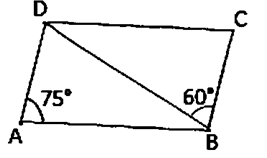Solution:
∠CBD = ∠ABD = 60° (Alternate interior angle)
In ∠BDC
∠A = ∠C = 75° (opposite sides and angles of a parallelogram are equal)
∠CBD + ∠C + ∠CDB = 180° (sum of all angles in a triangle is 180°)
60° + 75° + ∠CDB = 180°
∠CDB = 180° − 60° - 75°
∠CDB = 180° − 135°
∠CDB = 45°
Hence, the measure of ∠CDB and ∠ADB are 45°and 60° respectively.

Question 9:  In figure, ABCD is a parallelogram and E is the mid-point of side BC. If DE and AB when produced meet at F, prove that AF = 2AB.Solution:
In ΔBEF and ΔCED
∠BEF = ∠CED (Vertically opposite angle)
BE = CE (E is the mid-point of BC)
∠EBF = ∠ECD (Alternate interior angles)
ΔBEF ≅ ΔCED (By ASA congruence)
BF = CD (CPCT)
BF = CD
AF = AB + BF
AF = AB + AB
AF = 2AB
Hence proved

Question 10:  Which of the following statements are true (T) and which are false (F)?
(i) In a parallelogram, the diagonals are equal.
(ii) In a parallelogram, the diagonals bisect each other.
(iii) In a parallelogram, the diagonals intersect each other at right angles.
(iv) In any quadrilateral, if a pair of opposite sides is equal, it is a parallelogram.
(v) If all the angles of a quadrilateral are equal, it is a parallelogram.
(vi) If three sides of a quadrilateral are equal, it is a parallelogram.
(vii) If three angles of a quadrilateral are equal, it is a parallelogram.
(viii) If all the sides of a quadrilateral are equal, it is a parallelogram.
Solution:
(i) In a parallelogram, the diagonals are equal. : False
(ii) In a parallelogram, the diagonals bisect each other. : True
(iii) In a parallelogram, the diagonals intersect each other at right angles. : False
(iv) In any quadrilateral, if a pair of opposite sides is equal, it is a parallelogram. : False
(v) If all the angles of a quadrilateral are equal, it is a parallelogram. : True
(vi) If three sides of a quadrilateral are equal, it is a parallelogram. : False
(vii) If three angles of a quadrilateral are equal, it is a parallelogram. : False
(viii) If all the sides of a quadrilateral are equal, it is a parallelogram. : True

Exercise 14.3

Question 1: In a parallelogram ABCD, determine the sum of angles ∠C and ∠D.
Solution:
It is given that ABCD is a parallelogram,
∠C and ∠D are consecutive interior angles on the same side of the transversal CD.
∠C + ∠D = 180°

Question 2: In a parallelogram ABCD, if ∠B = 135°, determine the measures of its other angles.
Solution:
It is given that ABCD is a parallelogram,
∠B = 135°
∠A = ∠C
∠B = ∠D
∠A + ∠B = 180° (consecutive interior angles)
∠A + 135° = 180°
∠A = 45°
∠A = ∠C = 45°
∠B = ∠D = 135°
Hence, the measure of ∠A, ∠B, ∠C and ∠D is 45°, 135°, 45° and 135°.

Question 3:  ABCD is a square. AC and BD intersect at O. State the measure of ∠AOB.
Solution:
∠AOB = 90°
We know that, diagonals of a square bisect each other at right angle.

Question 4:  ABCD is a rectangle with ∠ABD = 40°. Determine ∠DBC.
Solution:
∠ABC = 90° (each angle of a rectangle is 90°)
It is given that ∠ABD = 40°
∠ABD + ∠DBC = 90°
40° + ∠DBC = 90°
∠DBC = 90° - 40°
∠DBC = 50°
Hence, the measure of ∠DBC is 50°.

Question 5:  The sides AB and CD of a parallelogram ABCD are bisected at E and F. Prove that EBFD is a parallelogram.
Solution:It is given that, ABCD is a parallelogram.
AB ∥ DC and AB = DC (sides of a parallelogram)
EB ∥ DF and 1/2 × AB = 1/2 × DC
EB ∥ DF and EB = DF (sides of a parallelogram)
EBFD is a parallelogram.

Question 6: P and Q are the points of trisection of the diagonal BD of a parallelogram ABCD. Prove that CQ is parallel to AP. Prove also that AC bisects PQ.Solution:
BD is a diagonal a parallelogram ABCD
OA = OC and OB = OD
P and Q are point of intersection of BD.
BP = PQ = QD
OB = OD are BP = QD
OB - BP = OD - QD
OP = OQ
OA = OC and OP = OQ
Diagonals of Quadrilateral APCQ bisect each other.
APCQ is a parallelogram
AP ∥ CQ
Hence Proved

Question 7: ABCD is a square. E, F, G and H are points on AB, BC, CD and DA respectively, such that AE = BF = CG = DH. Prove that EFGH is a square.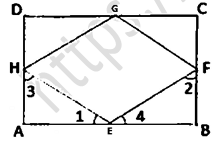Solution:
It is given that,
ABCD is a square
AE = BF = CG = DH
BE = CF = DG = AH
In ΔAEH and ΔBEF
AE = BF
∠A = ∠B
AH = BE
ΔAEH ≅ ΔBFE (by SAS congruency criterion)
∠1 = ∠2 (CPCT)
∠3 = ∠4 (CPCT)
∠1 + ∠3 = 90° _______(1)
∠2 + ∠4 = 90° _______(2)
∠1 + ∠3 + ∠2 + ∠4 = 90° + 90° (∠1 = ∠2 proved above)
∠1 + ∠4 + ∠1 + ∠4 = 180°
2∠1 + 2∠4 = 180°
2(∠1 + ∠4) = 180°
∠1 + ∠4 = (180°)/2
∠1 + ∠4 = 90°
∠HEF = 90°
∠GFE = ∠FGH = ∠GHE = 90°
EFGH is a Square.
Hence Proved

Question 8:  ABCD is a rhombus, EAFB is a straight line such that EA = AB = BF. Prove that ED and FC when produced meet at right angles.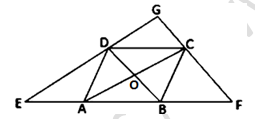Solution:
It is given that ABCD is a rhombus.
OA = OC, OB = OD
∠AOD = ∠COD = 90°
∠AOB = ∠COB = 90°
In ΔBDE,
A and O are mid-points of BE and BD respectively.
OA ∥ DE
OC ∥ DG
In ΔCFA,
B and O are mid-points of AF and AC respectively.
OB ∥ CF
OD ∥ GC
OC ∥ DG
OD ∥ GC
DOCG is a parallelogram
∠DGC = ∠DOC
∠DGC = 90°
Hence Proved

Question 9:  ABCD is a parallelogram, AD is produced to E so that DE = DC and EC produced meets AB produced in F. Prove that BF = BC.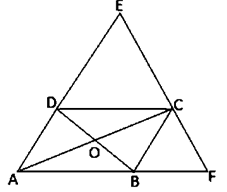Solution:
ABCD is a parallelogram.
DC = AB
DE = DC (Given)
In ∆EAF
By converse if mid-point theorem
DC||AB
DC||AF
D and C are the mid points of AE and FE.
DE = AD = BC = DC = AB
In ∆ACE
O is mid-point of AC
D is mid-point of AE
DO||EC and DO||EF
DB||EF and OB||CF
In ∆ACF
O is mid-point of AC
OB||CF (mid-point theorem)
B is mid-point of AF
AB = BF
BC = AB = BF
BC = BF
Hence proved

Exercise 14.4

Question 1: In a ΔABC, D, E and F are, respectively, the mid points of BC, CA and AB. If the lengths of sides AB, BC and CA are 7 cm, 8 cm and 9 cm, respectively, find the perimeter of ΔDEF.
Solution:
It is given that,
AB = 7 cm, BC = 8 cm, AC = 9 cm
In ∆ABC,
D, E and F are, respectively, the mid points of BC, CA and AB.
EF = 1/2 BC (Midpoint Theorem)
DF = 1/2 AC (Midpoint Theorem)
DE = 1/2 AB (Midpoint Theorem)
Perimeter of Triangle = Side + Side + Side
Perimeter of ∆DEF = DE + EF + DF
Perimeter of ∆DEF = 1/2AB + 1/2BC + 1/2AC
Perimeter of ∆DEF = 1/2 (AB + BC + AC)
Perimeter of ∆DEF = 1/2 (7 + 8 + 9)
Perimeter of ∆DEF = 1/2 (24)
Perimeter of ∆DEF = 12cm

Question 2: In a ΔABC, ∠A = 50°, ∠B = 60° and ∠C = 70°. Find the measures of the angles of the triangle formed by joining the mid-points of the sides of this triangle.
Solution: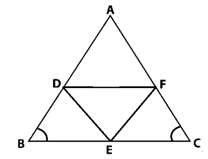In ΔABC,
D, E and F are mid points of AB, BC and AC
DE ∥ AC
DE = AC/2 (By Mid-point theorem)
CF = AC/2
DE = CF
DECF is a parallelogram.
∠C = ∠D = 70° (Opposite sides of a parallelogram)
Similarly,
ADEF is a parallelogram, ∠A = ∠E = 50°
BEFD is a parallelogram, ∠B = ∠F = 60°
Hence, the ∠D, ∠E and ∠F are 70°, 50° and 60°.

Question 3: In a triangle, P, Q and R are the mid points of sides BC, CA and AB respectively. If AC = 21 cm, BC = 29 cm and AB = 30 cm, find the perimeter of the quadrilateral ARPQ.
Solution: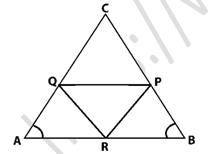In ΔABC,
R and P are mid points of AB and BC
RP ∥ AC ⇒ RP = AC/2 (By Mid-point Theorem)
RP ∥ AQ
RP = AQ (A pair of side is parallel and equal)
So, ARPQ is a parallelogram.
AR = AB/2
AR = 30/2
AR = 15 cm
AR = QP = 15 cm (Opposite sides are equal)
RP = AC/2
RP = 21/2
RP = 10.5 cm
RP = AQ = 10.5cm (Opposite sides are equal)
Perimeter of ARPQ = AR + QP + RP +AQ
Perimeter of ARPQ = 15 +15 +10.5 +10.5
Perimeter of ARPQ = 51
Hence, the perimeter of quadrilateral ARPQ is 51 cm.

Question 4: In a ΔABC median AD is produced to X such that AD = DX. Prove that ABXC is a parallelogram.
Solution: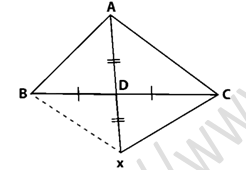It is given that, ABXC is a quadrilateral
BD = DC (Given)
Diagonals AX and BC bisect each other.
ABXC is a parallelogram.
Hence Proved

Question 5: In a ΔABC, E and F are the mid-points of AC and AB respectively. The altitude AP to BC intersects FE at Q. Prove that AQ = QP.
Solution: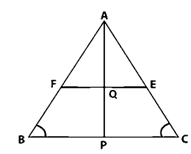It is given that,
In ΔABC
E and F are mid points of AC and AB
EF ∥ BC
EF = BC/2 (By mid-point theorem)
In ΔABP
F is the mid-point of AB, again by mid-point theorem
FQ ∥ BP
Q is the mid-point of AP
AQ = QP
Hence Proved

Question 6: In a ΔABC, BM and CN are perpendiculars from B and C respectively on any line passing through A. If L is the mid-point of BC, prove that ML = NL.
Solution: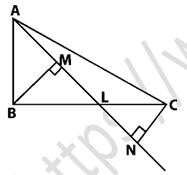Given that, BM and CN are perpendiculars from B and C.
In ΔBLM and ΔCLN
∠BML = ∠CNL (each 90°)
BL = CL (L is the mid-point)
∠MLB = ∠NLC (Vertically opposite angle)
ΔBLM ≅ ΔCLN (By ASA criterion)
LM = LN (CPCT)

Question 7: In figure, triangle ABC is a right-angled triangle at B. Given that AB = 9 cm, AC = 15 cm and D, E are the mid-points of the sides AB and AC respectively, calculate
(i) The length of BC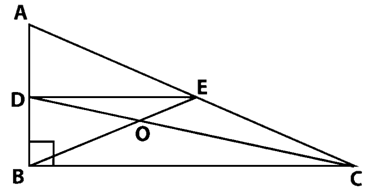Solution:
In ΔABC,
It is given that, ∠B = 90°, AB = 9 cm, AC = 15 cm
AC2 = AB2 + BC2 (By using Pythagoras theorem)
152 = 92 + AC2
AC2 = 225 – 81 = 144
BC = 12
AD = DB = AB/2 (D is the mid−point of AB)
AD = DB = 4.5 cm
D and E are mid-points of AB and AC
DE ∥ BC
DE = BC/2 (By mid−point theorem)
Area of ΔADE = 1/2 × 4.5 × 6
Hence, the area of ΔADE is 13.5 cm2

Question 8: In figure, M, N and P are mid-points of AB, AC and BC respectively. If MN = 3 cm, NP = 3.5 cm and MP = 2.5 cm, calculate BC, AB and AC.
Solution: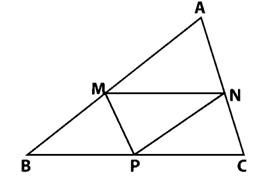It is given that, MN = 3 cm, NP = 3.5 cm and MP = 2.5 cm.
M and N are mid-points of AB and AC
By mid−point theorem, we have
MN||BC
MN = BC/2
MN = 3 cm (given)
BC = 2MN
BC = 6 cm
AC = 2MP
AC = 2(2.5)
AC = 5 cm
AB = 2 NP
AB = 2(3.5)
AB = 7 cm

Question 9: ABC is a triangle and through A, B, C lines are drawn parallel to BC, CA and AB respectively intersecting at P, Q and R. Prove that the perimeter of ΔPQR is double the perimeter of ΔABC.
Solution:ABCQ and ARBC are parallelograms.
BC = AQ and BC = AR
AQ = AR (A is the mid-point of QR)
B and C are the mid points of PR and PQ respectively.
AB =  PQ/2 (By mid−point theorem)
BC =  QR/2 (By mid−point theorem)
CA =  PR/2 (By mid−point theorem)
PQ = 2AB
QR = 2BC
PR = 2CA
PQ + QR + RP = 2 (AB + BC + CA)
Perimeter of ΔPQR = 2 (Perimeter of ΔABC)

Question 10:  In figure, BE ⊥ AC, AD is any line from A to BC intersecting BE in H. P, Q and R are respectively the mid-points of AH, AB and BC. Prove that ∠PQR = 90°.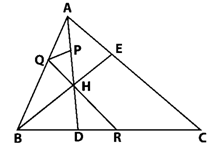Solution:
It is given that, BE ⊥ AC and P, Q and R are respectively mid-point of AH, AB and BC.
In ΔABC, Q and R are mid-points of AB and BC respectively.
By Mid-point theorem, we know
QR ∥ AC_________(i)
In ΔABH,
Q and P are the mid-points of AB and AH respectively
QP ∥ BH ________(ii)
But, BE ⊥ AC
From (i) and (ii) we have,
QP ⊥ QR
∠PQR = 90°
Hence Proved

Question 11: In figure, AB = AC and CP ∥ BA and AP is the bisector of exterior ∠CAD of ∆ABC. Prove that
(i) ∠PAC = ∠BCA.
(ii) ABCP is a parallelogram.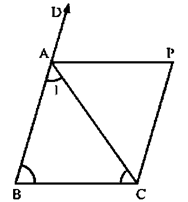Solution:
It is given that, AB = AC and CD ∥ BA and AP is the bisector of exterior ∠CAD of ΔABC
(i) It is given that,
AB = AC
∠ACB = ∠ABC (Opposite angles of equal sides)
2∠PAC = 2∠ACB
∠PAC = ∠ACB

(ii) It is given that,
∠PAC = ∠BCA
AP ∥ BC (Given)
CP ∥ BA (Given)
Thus, ABCP is a parallelogram.

Question 12:  ABCD is a kite having AB = AD and BC = CD. Prove that the figure found by joining the mid points of the sides, in order, is a rectangle.
Solution:It is given that, A kite ABCD having AB = AD and BC = CD. P, Q, R, S are the mid-points of sides AB, BC, CD and DA respectively. PQ, QR, RS and SP are joined.
In ∆ABC, P and Q are the mid-points of AB and BC respectively.
PQ ∥ AC
PQ = 1/2 AC_________(i)
In ∆ADC, R and S are the mid-points of CD and AD respectively.
RS ∥ AC
RS = 1/2 AC_________(ii)
From (i) and (ii) we get,
PQ ∥ RS
PQ = RS
A pair of opposite sides is equal and parallel.
Hence, PQRS is a parallelogram.
We shall prove that one angle of parallelogram PQRS is a right angle.
AP = AS________(iii) (P and S are midpoints of AB and AD)
∠1 = ∠2________(iv)
In ΔPBQ and ΔSDR, we have
PB = SD (1/2AD = 1/2AB)
BQ = DR (PB = SD)
PQ = SR (PQRS is a parallelogram)
ΔPBQ ≅ ΔSDR (SSS criterion of congruence)
∠3 = ∠4 (CPCT)
∠3 + ∠SPQ + ∠2 = 180°
∠1 + ∠PSR + ∠4 = 180°
∠3 + ∠SPQ + ∠2 = ∠1 + ∠PSR + ∠4
∠SPQ = ∠PSR (∠1 = ∠2 and ∠3 = ∠4)
Here, transversal PS cuts parallel lines SR and PQ at S and P respectively.
∠SPQ + ∠PSR = 180°
2∠SPQ = 180°
∠SPQ = 90° (∠PSR = ∠SPQ)
Thus, PQRS is a parallelogram such that ∠SPQ = 90°.
Hence, PQRS is a parallelogram.

Question 13:  Let ABC be an isosceles triangle in which AB = AC. If D, E, F be the mid points of the, sides BC, CA and AB respectively, show that the segment AD and EF bisect each other at right angles.
Solution:It is given that, D, E and F is mid-points of sides BC, CA and AB respectively.
ABC is a Isosceles triangle
AB ∥ DE and AC ∥ DF
AF ∥ DE and AE ∥ DF
So, ABDE is a parallelogram.
AF = DE
(1 )/2AB = DE
AE = DF
(1 )/2 AC = DF
DE = DF (AB = AC)
AE = AF = DE = DF
ABDF is a rhombus.
Hence, AD and FE bisect each other at right angle.

Question 14:  ABC is a triangle. D is a point on AB such that AD = 1/4 AB and E is a point on AC such that AE = 1/4 AC. Prove that DE = 1/4BC.
Solution:By construction we know that, P and Q be the mid-points of AB and AC respectively.
In ∆ABC,
PQ || BC
PQ = 1/2BC________(i) (by mid-point theorem)
In ΔAPQ,
D and E are the mid-points of AP and AQ respectively.
DE || PQ
DE = 1/2PQ ________(ii) (by mid-point theorem)
From equation (i) and (ii) we get,
DE = 1/2PQ
DE = 1/2 (1/2 BC)
DE = 1/2BC
Hence proved

Question 15: In Figure, ABCD is a parallelogram in which P is the mid-point of DC and Q is a point on AC such that CQ = (1 )/2 AC. If PQ produced meets BC at R, prove that R is a mid-point of BC.Solution:Join B and D
AC and BD intersect each other at O.
ABCD is a parallelogram
OC = 1/2AC
CQ = 1/2 (1/2 AC)
CQ = 1/2OC
ΔDCO, P and Q are mid points of DC and OC respectively.
PQ ∥ DO
In ΔCOB, Q is the mid-point of OC
QR ∥ OB
Hence, R is the mid-point of BC.

Question 16: In figure, ABCD and PQRC are rectangles and Q is the mid-point of AC. Prove that
(i) DP = PC
(ii) PR = 1/2 ACSolution:
Q is the mid-point of AC
Thus, P is the mid-point of DC.
DP = DC (By mid-point theorem)

(ii) Similarly, R is the mid-point of BC
PR = 1/2 BD
Diagonal of rectangle are equal, BD = AC
PR = 1/2 AC

Question 17: ABCD is a parallelogram; E and f are the mid-points of AB and CD respectively. GH is any line intersecting AD, EF and BC at G, P and H respectively. Prove that GP = PH.
Solution:It is given that, E and F are mid-points of AB and CD respectively.
ABCD is a parallelogram
AE = BE = 1/2AB
CF = DF = 1/2CD
AB = CD
1/2AB = 1/2CD
BE = CF
Thus, BEFC is a parallelogram
BC ∥ EF and BE = PH_______(i)
BC ∥ EF
AD ∥ EF (ABCD is a parallelogram)
Thus, AEFD is a parallelogram.
AE = GP
But E is the mid-point of AB.
So, AE = BF
Thus, GP = PH.

Question 18:  BM and CN are perpendiculars to a line passing through the vertex A of triangle ABC. If L is the mid-point of BC, prove that LM = LN.
Solution: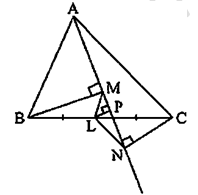BM and CN are perpendiculars to a line passing through the vertex A of triangle ABC.
We have,
BL = LC   [As L is the given mid-point of BC]
Using the intercept theorem, we get
MS = SN
In ΔMLS and ΔLSN
MS = SN (Proved above)
∠LSM = ∠LSN (each 90°)
SL = LS (common)
ΔMLS ≅ ΔLSN (SAS Congruency Theorem)
LM = LN (CPCT)

Question 19:  Show that, the line segments joining the mid-points of opposite sides of a quadrilateral bisects each other.
Solution: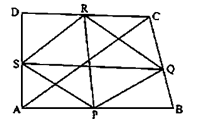Let us assumed that,
ABCD is a quadrilateral in which P, Q, R and S are mid-points of sides AB, BC, CD and DA respectively.
SP ∥ BD
SP = 1/2 BD________(i) (mid-point theorem)
In ΔBCD
QR ∥ BD
QR = 1/2 BD________ (ii)
From equations (i) and (ii), we get
SP ∥ QR
SP = QR
Hence PR and QS bisect each other.

Question 20:  Fill in the blanks to make the following statements correct:
(i) The triangle formed by joining the mid-points of the sides of an isosceles triangle is ____________.
(ii) The triangle formed by joining the mid-points of the sides of a right triangle is ____________.
(iii) The figure formed by joining the mid-points of consecutive sides of a quadrilateral is ____________.
Solution:
(i) The triangle formed by joining the mid-points of the sides of an isosceles triangle is Isosceles.
(ii) The triangle formed by joining the mid-points of the sides of a right triangle is Right triangle.
(iii) The figure formed by joining the mid-points of consecutive sides of a quadrilateral is Parallelogram.

Exercise VSAQs

Question 1: In a parallelogram ABCD, write the sum of angles A and B.
Solution:
It is given that ABCD parallelogram
∠A + ∠B = 180° (Adjacent angles of a parallelogram are supplementary)

Question 2: In a parallelogram ABCD, if ∠D = 115°, then write the measure of ∠A.
Solution:
It is given that ABCD parallelogram,
∠D = 115°
Since, ∠A and ∠D are adjacent angles of parallelogram.
∠A + ∠D = 180° (Adjacent angles of a parallelogram are supplementary)
∠A = 180° – 115°
∠A = 65°
Hence, the measure of ∠A is 65°.

Question 3: PQRS is a square such that PR and SQ intersect at O. State the measure of ∠POQ.
Solution:
It is given that, PQRS is a square.
Such that PR and SQ intersect at O
∠POQ = 90° (Diagonals of a square bisects each other at 90°)

Question 4: In a quadrilateral ABCD, bisectors of angles A and B intersect at O such that ∠AOB = 75°, then write the value of ∠C + ∠D.
Solution:
It is given that, ∠AOB = 75°
In a quadrilateral ABCD, bisectors of angles A and B intersect at O, then
∠AOB =  1/2 (∠ADC + ∠ABC)
∠AOB =  1/2 (∠D + ∠C)
75° =  1/2 (∠D + ∠C)
∠C + ∠D = 150°

Question 5: The diagonals of a rectangle ABCD meet at O. If ∠BOC = 44°, find ∠OAD.
Solution:
It is given that, ABCD is a rectangle
∠BOC = 44°
∠AOD = ∠BOC (vertically opposite angles)
∠AOD = ∠BOC (each 44°)
∠OAD = ∠ODA (Angles on same sides are equal)
OD = OA
∠OAD = 1/2 (180° – 44°) (Sum of all the angles of a triangle is 180°)
Hence, the measure of is ∠OAD = 68°

Question 6: If PQRS is a square, then write the measure of ∠SRP.
Solution:
PQRS is a square.
∠SRP =  1/2 (90°)
∠SRP = 45°

Question 7: If ABCD is a rectangle with ∠BAC = 32°, find the measure of ∠DBC.
Solution:
It is given that, ABCD is a rectangle and ∠BAC = 32°
AO = BO
∠DBA = ∠BAC = 32° (Angles on same side)
∠DBC + ∠DBA = 90° (each angle of a rectangle is 90°)
∠DBC + 32° = 90°
∠DBC = 90° - 32°
∠DBC = 58°

Question 8: If ABCD is a rhombus with ∠ABC = 56°, find the measure of ∠ACD.
Solution:
In a rhombus ABCD,
∠ABC = 56°
∠BCD = 2 (∠ACD) (Diagonals of a rhombus bisect the interior angles)
∠ACD =  1/2 (∠BCD) ______(1)
∠BCD + ∠ABC = 180° (angles of a rhombus are supplementary)
∠BCD = 180° – 56°
∠BCD = 124°
Put the value of ∠BCD in equation in (1)
∠ACD = 1/2 × 124°
∠ACD = 62°

Question 9: The perimeter of a parallelogram is 22 cm. If the longer side measure 6.5 cm, what is the measure of shorter side?
Solution:
It is given that, Perimeter of a parallelogram = 22 cm.
Longer side of a parallelogram = 6.5 cm
Let x be the shorter side
Perimeter = 2(x + 6.5)
22 = 2x + 13
2x = 22 – 13
2x = 9
x = 4.5
Hence, the measure of shorter side is 4.5 cm.

Question 10: If the angles of a quadrilateral are in the ratio 3:5:9:13, then find the measure of the smallest angle.
Solution:
It is given that, Angles of a quadrilateral are in the ratio 3 : 5 : 9 : 13.
Let the sides are
A = 3x
B = 5x
C = 9x
D = 13x
A + B + C + D = 360° (sum of all the angles of a quadrilateral is 360°)
3x + 5x + 9x + 13x = 360°
30 x = 360°
x = 12°
Shortest angle is 3 × 12° = 36°
Hence, the measure of smallest angle is 36°.